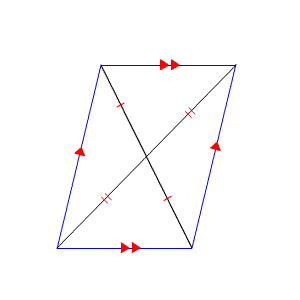# Diagonals of Parallelograms

There is a theorem that if a quadrilateral is a parallelogram , then the diagonals bisect each other.The converse of this theorem is also true, that is, if the diagonals of a quadrilateral bisect each other, then the quadrilateral is a parallelogram.